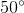# ISEE Lower Level Quantitative : Shape Properties

## Example Questions

← Previous 1 3

### Example Question #1 : Understand Categories And Subcategories Of Two Dimensional Figures: Ccss.Math.Content.5.G.B.3

Which of the following shapes is NOT a quadrilateral?

Square

Triangle

Rectangle

Kite

Rhombus

Triangle

Explanation:

A quadrilateral is any two-dimensional shape withsides. The only shape listed that does not havesides is a triangle.

### Example Question #1 : Understand Categories And Subcategories Of Two Dimensional Figures: Ccss.Math.Content.5.G.B.3

What is the main difference between a square and a rectangle?

Their color

Their angle measurments

The number of sides they each have

Their side lengths

The sum of their angles

Their side lengths

Explanation:

The only difference between a rectangle and a square is their side lengths. A square has to haveequal side lengths, but the opposite side lengths of a rectangle only have to be equal.

### Example Question #1 : Understand Categories And Subcategories Of Two Dimensional Figures: Ccss.Math.Content.5.G.B.3

What two shapes can a square be classified as?

Trapezoid and Rhombus

Rectangle and Rhombus

Trapezoid and Triangle

Rectangle and Triangle

Rhombus and Triangle

Rectangle and Rhombus

Explanation:

A square can also be a rectangle and a rhombus because a rectangle has to have at leastsets of equal side lengths and a rhombus has to haveequal side lengths, like a square, and at leastsets of equal angles.

### Example Question #2 : Understand Categories And Subcategories Of Two Dimensional Figures: Ccss.Math.Content.5.G.B.3

What is the main difference between a triangle and a rectangle?

The volume

The color

The area

The number of sides

The length of the sides

The number of sides

Explanation:

Out of the choices given, the only characteristic used to describe shapes is the number of sides. A triangle hassides and a rectangle hassides.

### Example Question #4 : Understand Categories And Subcategories Of Two Dimensional Figures: Ccss.Math.Content.5.G.B.3

Which two shapes have to haveright angles?

Square and Parallelogram

Square and Rectangle

Rectangle and Parallelogram

Square and Rhombus

Rectangle and Rhombus

Square and Rectangle

Explanation:

By definition, the only two quadrilaterals that have to haveright angles, are the square and the rectangle.

### Example Question #831 : Geometry

Which of the shapes is NOT a quadrilateral?

Hexagon

Trapezoid

Rectangle

Square

Rhombus

Hexagon

Explanation:

A quadrilateral is asided shape. The only shape listed that does not havesides is a hexagon, which hassides.

### Example Question #6 : Understand Categories And Subcategories Of Two Dimensional Figures: Ccss.Math.Content.5.G.B.3

Which of the following shapes is NOT a parallelogram?

Rectangle

Square

Rhombus

Kite

Kite

Explanation:

A rectangle, square, and rhombus can all be classified as a parallelogram because each shape has opposite side lengths that are equal. A kite does not.

### Example Question #52 : Geometry

What is the difference between a trapezoid and a isosceles trapezoid?

An isosceles trapezoid has to have equal side lengths

A trapezoid has to have equal side lengths

An isosceles trapezoid has to have equal base angles

A trapezoid has to have equal base angles

There is no difference between them

An isosceles trapezoid has to have equal base angles

Explanation:

By definition, an isosceles trapezoid has to haveequal base angles, but a trapezoid does not have to have equal angles.

### Example Question #8 : Understand Categories And Subcategories Of Two Dimensional Figures: Ccss.Math.Content.5.G.B.3

Which shape is NOT a quadrilateral?

Rhombus

Trapezoid

Rectangle

Circle

Kite

Circle

Explanation:

A quadrilateral has to havesides, a circle does not have any sides.

### Example Question #9 : Right Triangles

What is the main difference between a right triangle and an isosceles triangle?

A right triangle has to have aangle and an isosceles triangle has to haveequal, base angles.

A right triangle has to have aangle and an isosceles triangle has to haveequal, base angles.

An isosceles triangle has to have aangle and a right triangle has to haveequal, base angles.

A right triangle has to have aangle and an isosceles triangle has to haveequal, base angles.

A right triangle has to have aangle and an isosceles triangle has to haveequal, base angles.

A right triangle has to have aangle and an isosceles triangle has to haveequal, base angles.
By definition, a right triangle has to have one right angle, or aangle, and an isosceles triangle hasequal base angles and two equal side lengths.